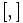# Second derived subgroup

(diff) ← Older revision | Latest revision (diff) | Newer revision → (diff)
The second derived subgroup of a group$G$, denoted$\! G''$, is defined in the following equivalent ways:
1. It is the subgroup generated by all elements of the form$[[x,y],[z,w]]$, where$[,]$ denotes the commutator and$x,y,z,w \in G$.
2. It is the normal closure of the subgroup generated by all elements of the form$[[x,y],[z,w]]$ where$[,]$ denotes the commutator and$x,y,z,w \in G$.
3. It is the derived subgroup of the derived subgroup of$G$.
4. It is the intersection of all subgroups$H$ of$G$ for which$G/H$ is a metabelian group, i.e., a solvable group of derived length two.